# Lecture Slides for INTRODUCTION TO Machine Learning 2

• Slides: 37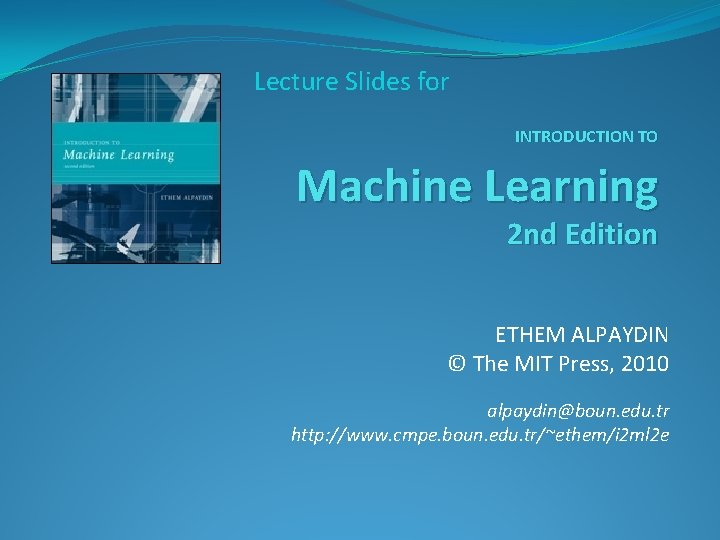Lecture Slides for INTRODUCTION TO Machine Learning 2 nd Edition ETHEM ALPAYDIN © The MIT Press, 2010 [email protected] edu. tr http: //www. cmpe. boun. edu. tr/~ethem/i 2 ml 2 e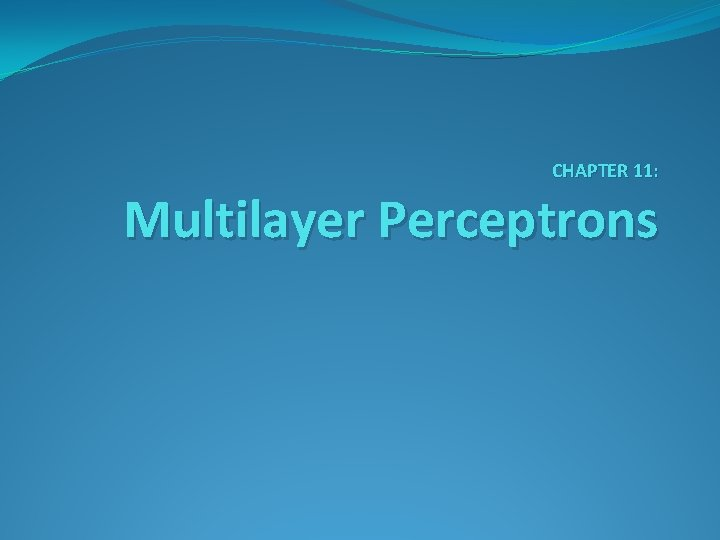CHAPTER 11: Multilayer Perceptrons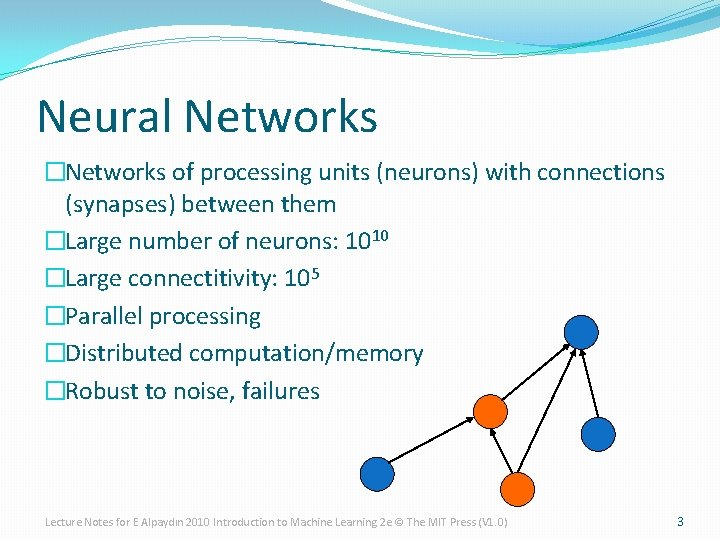Neural Networks �Networks of processing units (neurons) with connections (synapses) between them �Large number of neurons: 1010 �Large connectitivity: 105 �Parallel processing �Distributed computation/memory �Robust to noise, failures Lecture Notes for E Alpaydın 2010 Introduction to Machine Learning 2 e © The MIT Press (V 1. 0) 3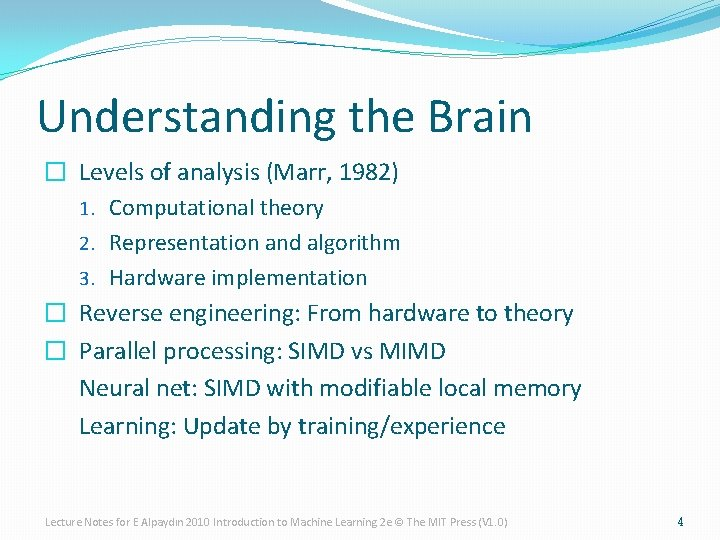Understanding the Brain � Levels of analysis (Marr, 1982) 1. Computational theory 2. Representation and algorithm 3. Hardware implementation � Reverse engineering: From hardware to theory � Parallel processing: SIMD vs MIMD Neural net: SIMD with modifiable local memory Learning: Update by training/experience Lecture Notes for E Alpaydın 2010 Introduction to Machine Learning 2 e © The MIT Press (V 1. 0) 4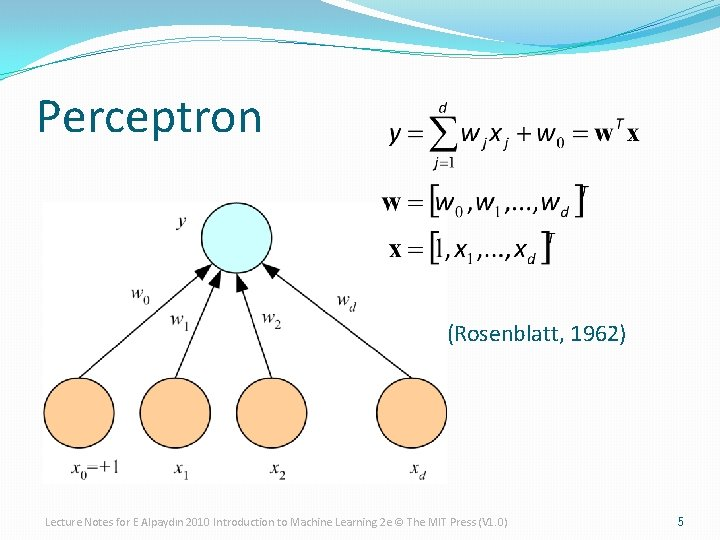Perceptron (Rosenblatt, 1962) Lecture Notes for E Alpaydın 2010 Introduction to Machine Learning 2 e © The MIT Press (V 1. 0) 5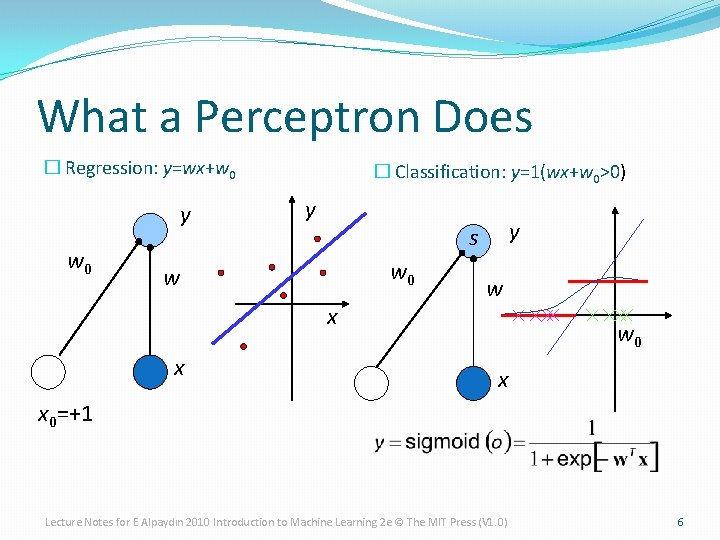What a Perceptron Does � Regression: y=wx+w 0 y w 0 � Classification: y=1(wx+w 0>0) y w 0 w x x y s w w 0 x x 0=+1 Lecture Notes for E Alpaydın 2010 Introduction to Machine Learning 2 e © The MIT Press (V 1. 0) 6Regression: K Outputs Classification: Lecture Notes for E Alpaydın 2010 Introduction to Machine Learning 2 e © The MIT Press (V 1. 0) 7Training �Online (instances seen one by one) vs batch (whole sample) learning: �No need to store the whole sample �Problem may change in time �Wear and degradation in system components �Stochastic gradient-descent: Update after a single pattern �Generic update rule (LMS rule): Lecture Notes for E Alpaydın 2010 Introduction to Machine Learning 2 e © The MIT Press (V 1. 0) 8Training a Perceptron: Regression � Regression (Linear output): Lecture Notes for E Alpaydın 2010 Introduction to Machine Learning 2 e © The MIT Press (V 1. 0) 9Classification �Single sigmoid output �K>2 softmax outputs Lecture Notes for E Alpaydın 2010 Introduction to Machine Learning 2 e © The MIT Press (V 1. 0) 10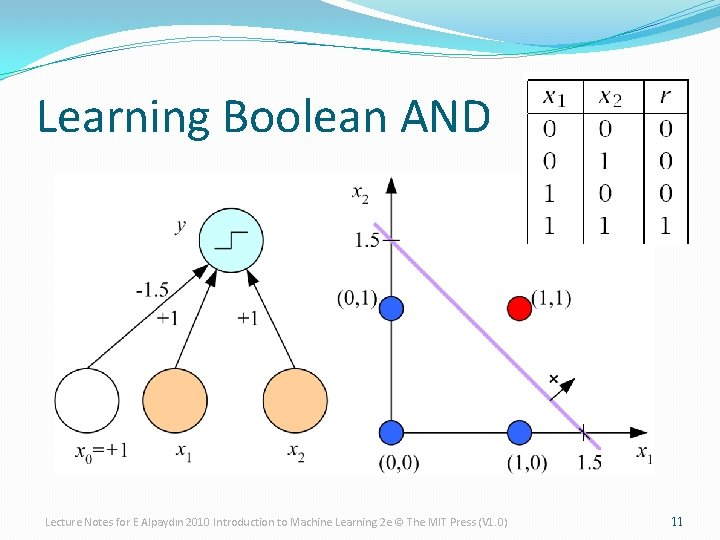Learning Boolean AND Lecture Notes for E Alpaydın 2010 Introduction to Machine Learning 2 e © The MIT Press (V 1. 0) 11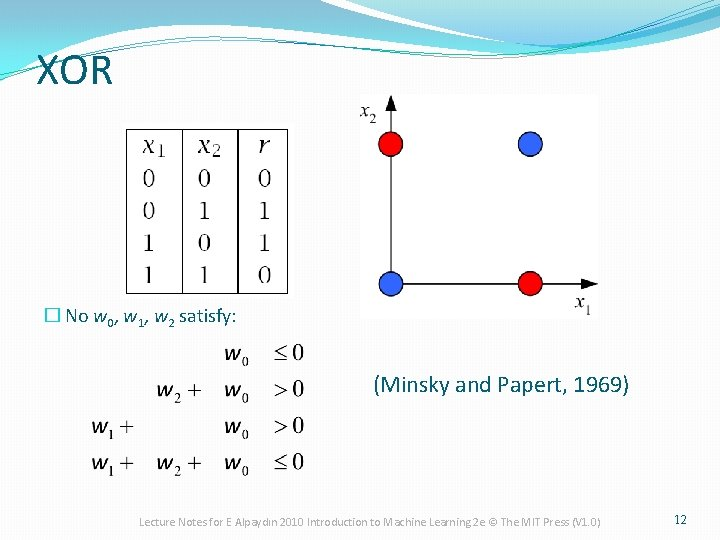XOR � No w 0, w 1, w 2 satisfy: (Minsky and Papert, 1969) Lecture Notes for E Alpaydın 2010 Introduction to Machine Learning 2 e © The MIT Press (V 1. 0) 12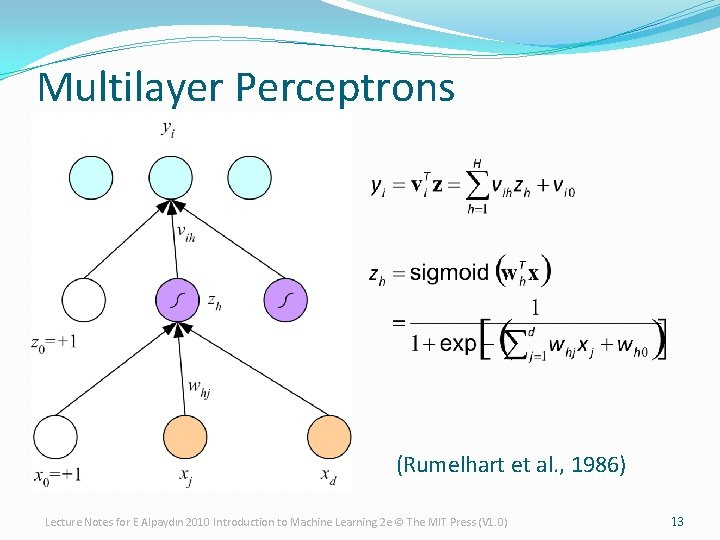Multilayer Perceptrons (Rumelhart et al. , 1986) Lecture Notes for E Alpaydın 2010 Introduction to Machine Learning 2 e © The MIT Press (V 1. 0) 13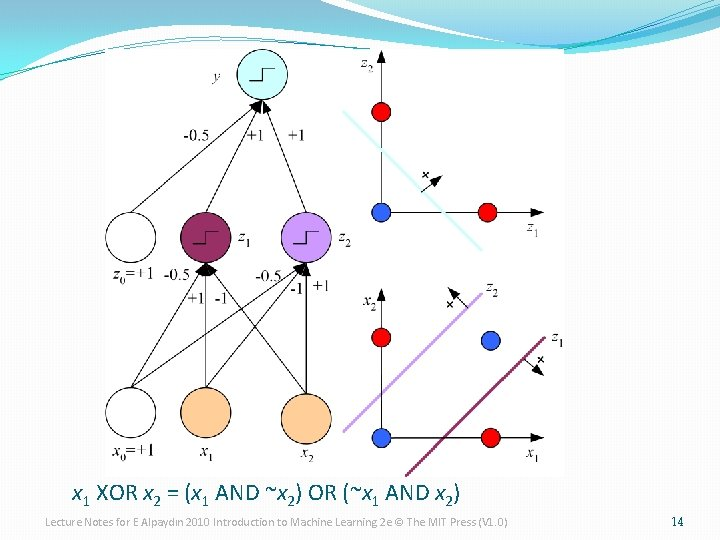x 1 XOR x 2 = (x 1 AND ~x 2) OR (~x 1 AND x 2) Lecture Notes for E Alpaydın 2010 Introduction to Machine Learning 2 e © The MIT Press (V 1. 0) 14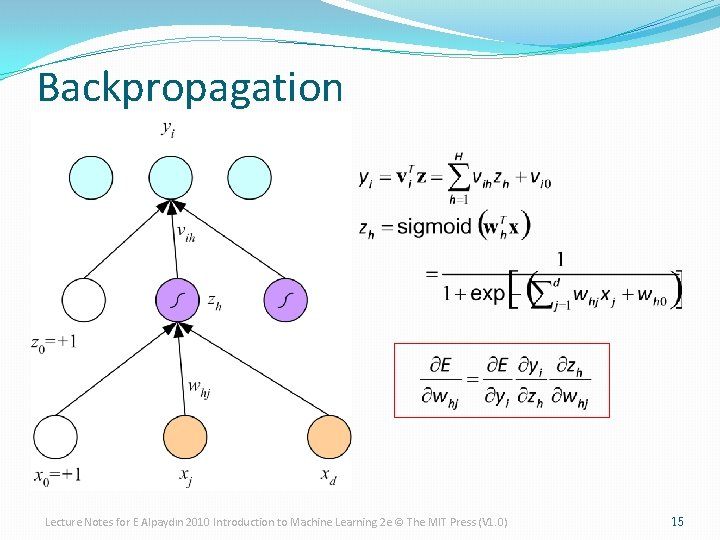Backpropagation Lecture Notes for E Alpaydın 2010 Introduction to Machine Learning 2 e © The MIT Press (V 1. 0) 15Regression Backward Forward x Lecture Notes for E Alpaydın 2010 Introduction to Machine Learning 2 e © The MIT Press (V 1. 0) 16Regression with Multiple Outputs y i vih zh whj xj Lecture Notes for E Alpaydın 2010 Introduction to Machine Learning 2 e © The MIT Press (V 1. 0) 17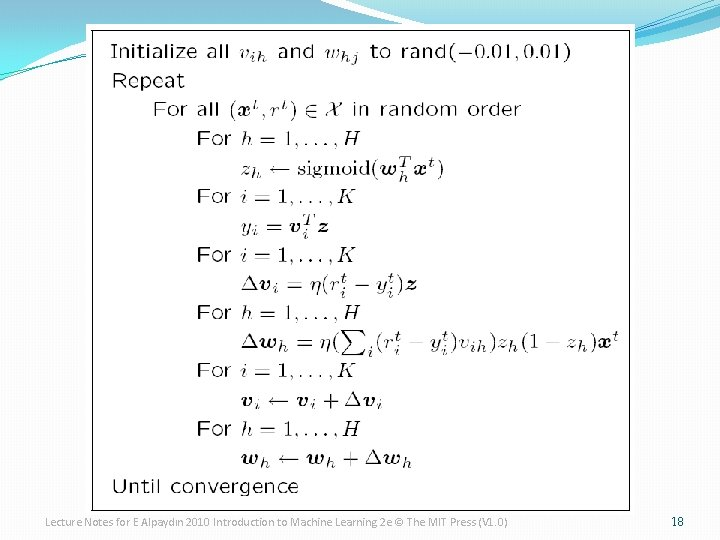Lecture Notes for E Alpaydın 2010 Introduction to Machine Learning 2 e © The MIT Press (V 1. 0) 18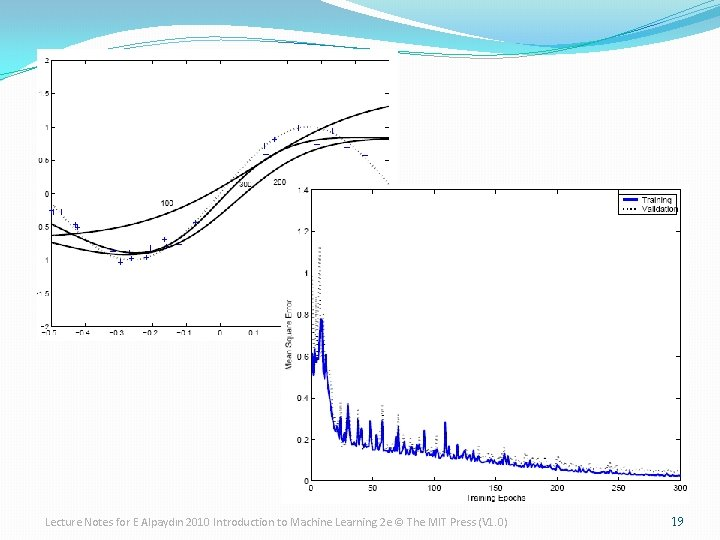Lecture Notes for E Alpaydın 2010 Introduction to Machine Learning 2 e © The MIT Press (V 1. 0) 19whx+w 0 zh Lecture Notes for E Alpaydın 2010 Introduction to Machine Learning 2 e © The MIT Press (V 1. 0) v h zh 20Two-Class Discrimination �One sigmoid output yt for P(C 1|xt) and P(C 2|xt) ≡ 1 -yt Lecture Notes for E Alpaydın 2010 Introduction to Machine Learning 2 e © The MIT Press (V 1. 0) 21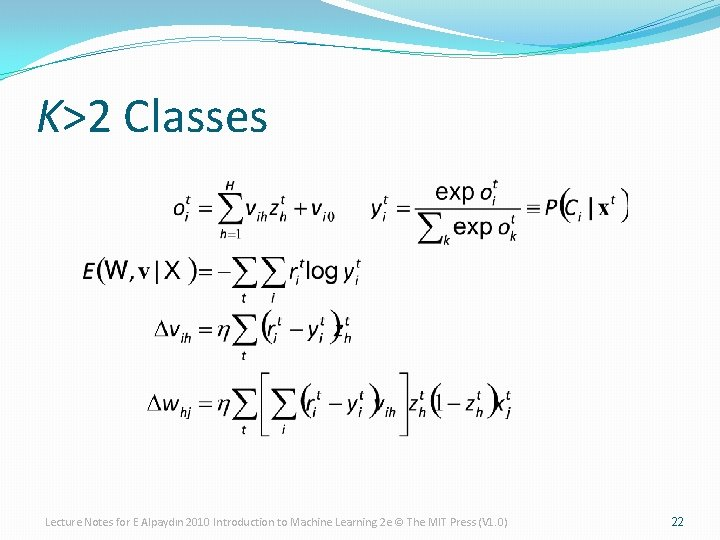K>2 Classes Lecture Notes for E Alpaydın 2010 Introduction to Machine Learning 2 e © The MIT Press (V 1. 0) 22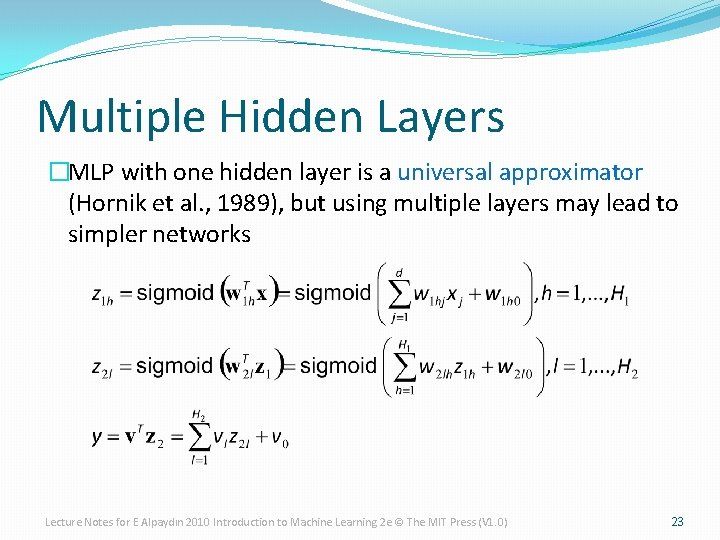Multiple Hidden Layers �MLP with one hidden layer is a universal approximator (Hornik et al. , 1989), but using multiple layers may lead to simpler networks Lecture Notes for E Alpaydın 2010 Introduction to Machine Learning 2 e © The MIT Press (V 1. 0) 23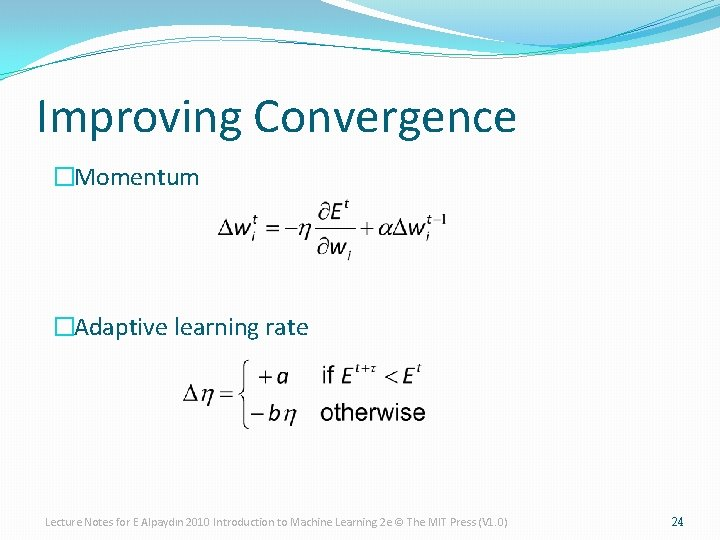Improving Convergence �Momentum �Adaptive learning rate Lecture Notes for E Alpaydın 2010 Introduction to Machine Learning 2 e © The MIT Press (V 1. 0) 24Overfitting/Overtraining Number of weights: H (d+1)+(H+1)K Lecture Notes for E Alpaydın 2010 Introduction to Machine Learning 2 e © The MIT Press (V 1. 0) 25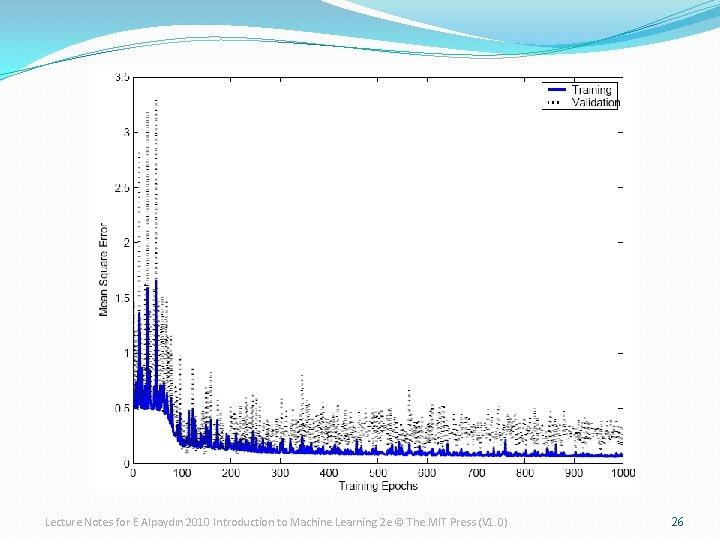Lecture Notes for E Alpaydın 2010 Introduction to Machine Learning 2 e © The MIT Press (V 1. 0) 26Structured MLP (Le Cun et al, 1989) Lecture Notes for E Alpaydın 2010 Introduction to Machine Learning 2 e © The MIT Press (V 1. 0) 27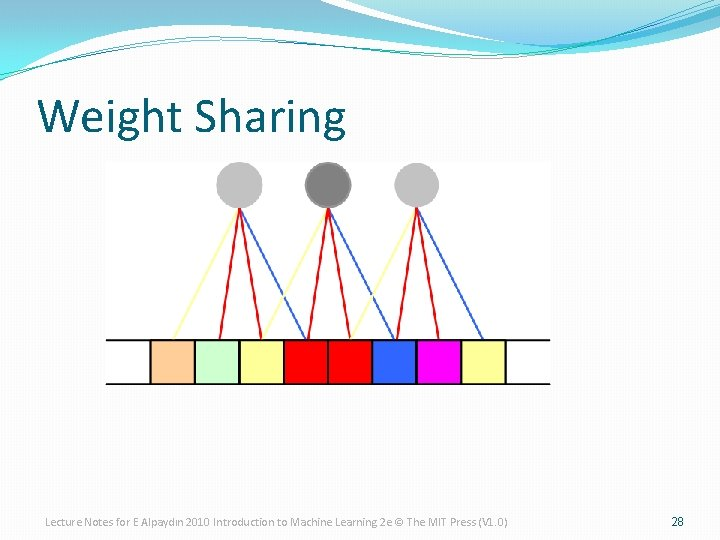Weight Sharing Lecture Notes for E Alpaydın 2010 Introduction to Machine Learning 2 e © The MIT Press (V 1. 0) 28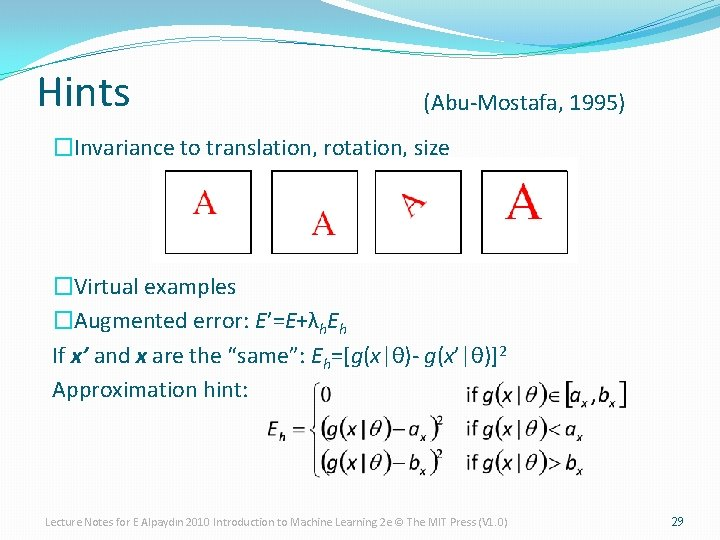Hints (Abu-Mostafa, 1995) �Invariance to translation, rotation, size �Virtual examples �Augmented error: E’=E+λh. Eh If x’ and x are the “same”: Eh=[g(x|θ)- g(x’|θ)]2 Approximation hint: Lecture Notes for E Alpaydın 2010 Introduction to Machine Learning 2 e © The MIT Press (V 1. 0) 29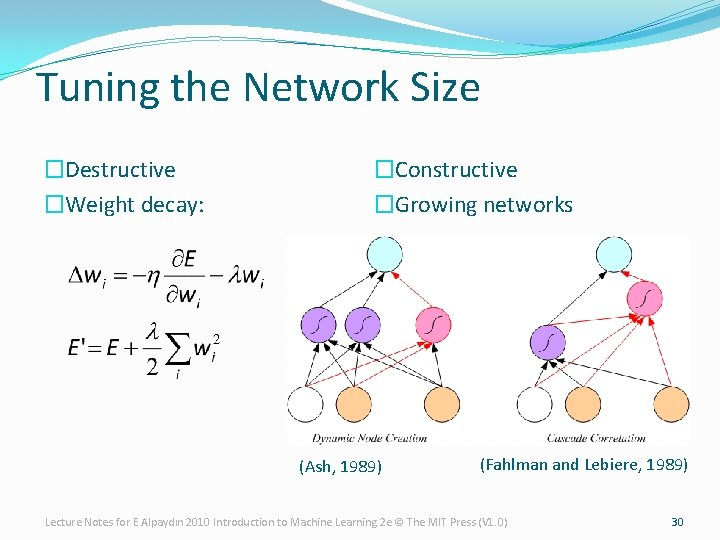Tuning the Network Size �Destructive �Weight decay: �Constructive �Growing networks (Ash, 1989) (Fahlman and Lebiere, 1989) Lecture Notes for E Alpaydın 2010 Introduction to Machine Learning 2 e © The MIT Press (V 1. 0) 30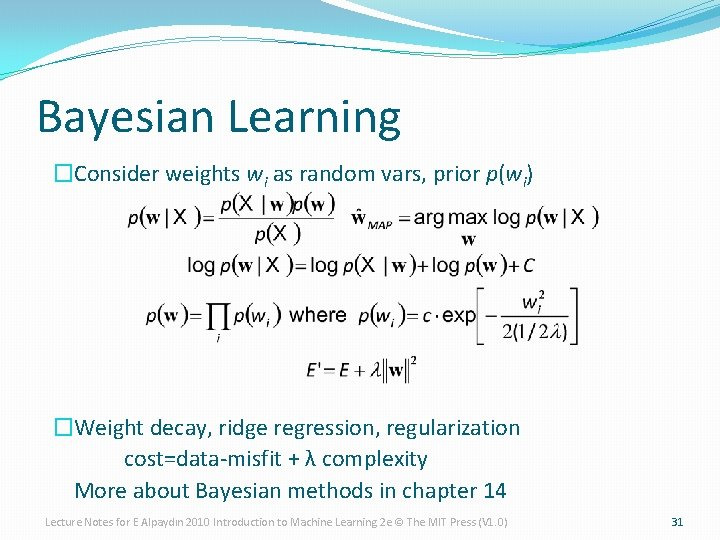Bayesian Learning �Consider weights wi as random vars, prior p(wi) �Weight decay, ridge regression, regularization cost=data-misfit + λ complexity More about Bayesian methods in chapter 14 Lecture Notes for E Alpaydın 2010 Introduction to Machine Learning 2 e © The MIT Press (V 1. 0) 31Dimensionality Reduction Lecture Notes for E Alpaydın 2010 Introduction to Machine Learning 2 e © The MIT Press (V 1. 0) 32Lecture Notes for E Alpaydın 2010 Introduction to Machine Learning 2 e © The MIT Press (V 1. 0) 33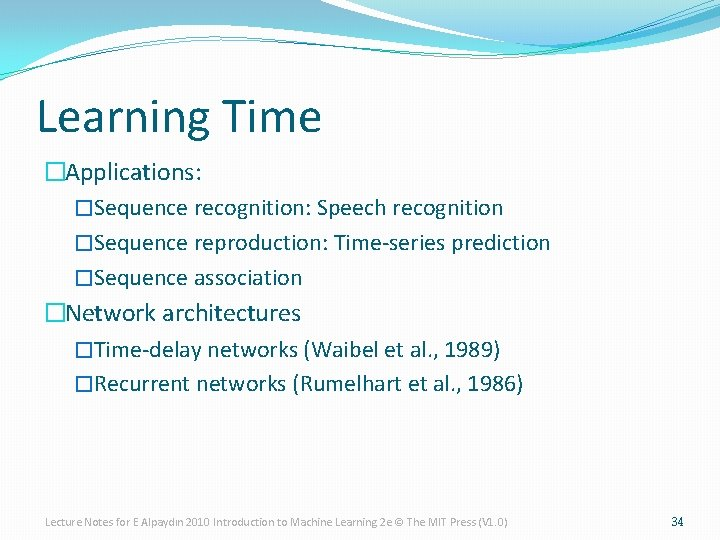Learning Time �Applications: �Sequence recognition: Speech recognition �Sequence reproduction: Time-series prediction �Sequence association �Network architectures �Time-delay networks (Waibel et al. , 1989) �Recurrent networks (Rumelhart et al. , 1986) Lecture Notes for E Alpaydın 2010 Introduction to Machine Learning 2 e © The MIT Press (V 1. 0) 34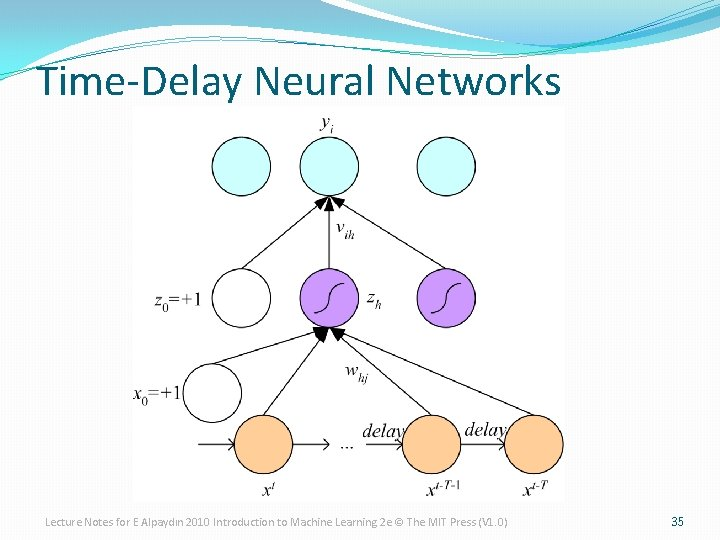Time-Delay Neural Networks Lecture Notes for E Alpaydın 2010 Introduction to Machine Learning 2 e © The MIT Press (V 1. 0) 35Recurrent Networks Lecture Notes for E Alpaydın 2010 Introduction to Machine Learning 2 e © The MIT Press (V 1. 0) 36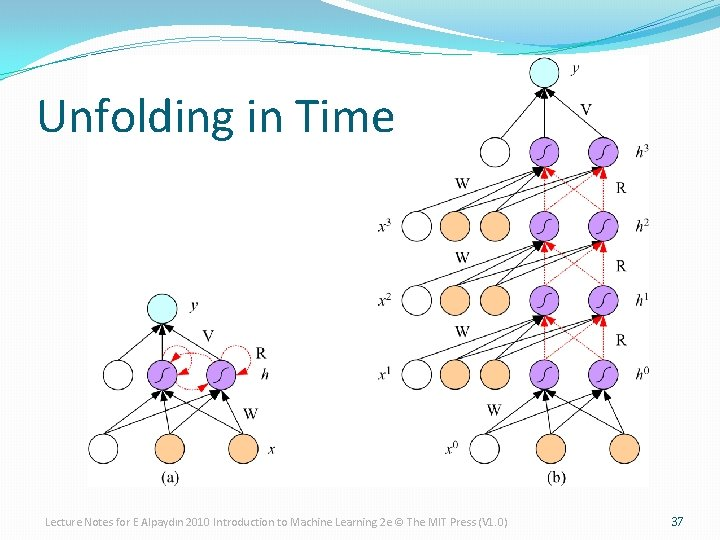Unfolding in Time Lecture Notes for E Alpaydın 2010 Introduction to Machine Learning 2 e © The MIT Press (V 1. 0) 37